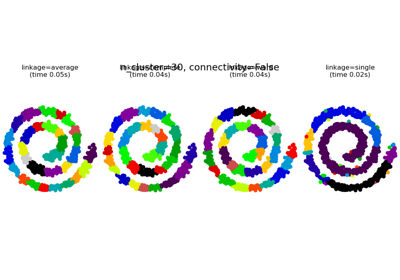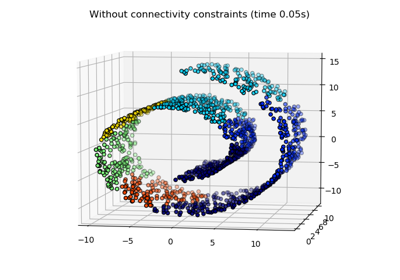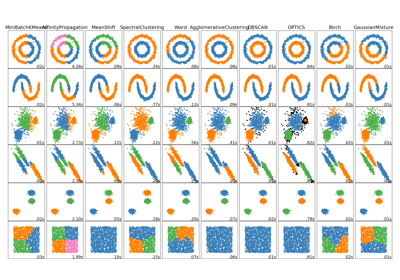# sklearn.neighbors.kneighbors_graph¶

sklearn.neighbors.kneighbors_graph(X, n_neighbors, mode='connectivity', metric='minkowski', p=2, metric_params=None, include_self=False, n_jobs=None)[source]

Computes the (weighted) graph of k-Neighbors for points in X

Read more in the User Guide.

Parameters
Xarray-like of shape (n_samples, n_features) or BallTree

Sample data, in the form of a numpy array or a precomputed BallTree.

n_neighborsint

Number of neighbors for each sample.

mode{‘connectivity’, ‘distance’}, optional

Type of returned matrix: ‘connectivity’ will return the connectivity matrix with ones and zeros, and ‘distance’ will return the distances between neighbors according to the given metric.

metricstring, default ‘minkowski’

The distance metric used to calculate the k-Neighbors for each sample point. The DistanceMetric class gives a list of available metrics. The default distance is ‘euclidean’ (‘minkowski’ metric with the p param equal to 2.)

pint, default 2

Power parameter for the Minkowski metric. When p = 1, this is equivalent to using manhattan_distance (l1), and euclidean_distance (l2) for p = 2. For arbitrary p, minkowski_distance (l_p) is used.

metric_paramsdict, optional

additional keyword arguments for the metric function.

include_selfbool or ‘auto’, default=False

Whether or not to mark each sample as the first nearest neighbor to itself. If ‘auto’, then True is used for mode=’connectivity’ and False for mode=’distance’.

n_jobsint or None, optional (default=None)

The number of parallel jobs to run for neighbors search. None means 1 unless in a joblib.parallel_backend context. -1 means using all processors. See Glossary for more details.

Returns
Asparse graph in CSR format, shape = [n_samples, n_samples]

A[i, j] is assigned the weight of edge that connects i to j.

Examples

>>> X = [, , ]
>>> from sklearn.neighbors import kneighbors_graph
>>> A = kneighbors_graph(X, 2, mode='connectivity', include_self=True)
>>> A.toarray()
array([[1., 0., 1.],
[0., 1., 1.],
[1., 0., 1.]])


## Examples using sklearn.neighbors.kneighbors_graph¶